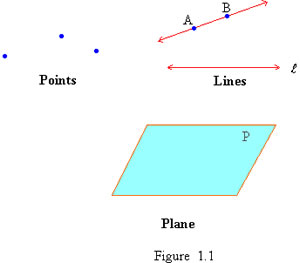# Difference between Point, Line and Plane

##### Key Difference: A point is a dot that denotes a location that has been marked on an infinite space or plane surface. A line is considered to be one-dimensional and was introduced to represent straight objects with no width and depth. A plane is a two-dimensional flat surface that is indefinitely large with zero thickness.The point, line and plane are all terms that are commonly found in mathematics. These make up the basic frame work of geometry and are a huge part of the Euclidean geometry. Euclidean geometry is a mathematical system that has been named in honor of Alexandrian Greek mathematician Euclid. Although much of Euclidean’s results were previously discovered by other mathematicians, he was the first one to assume a small set of intuitively appealing axioms, and deducing many other propositions (theorems) from these.

Point, line and plane are considered to be undefined terms of geometry because they are not formally defined. When we define a term, it is usually using simpler words to describe the term. However, a point, line and plane are considered as already simplified terms. All other geometric concepts are built on the point, line and plane. However, let’s try to understand these three undefined terms.

A point is a dot that does not denote a thing but instead, a position. The point represents a location that has been marked in the infinite space or on a plane surface. A point can be a dot of any size, but it does not have any length, width or thickness. This is because it is representing a place and not a thing.

Points are named using an upper-case single letter such as A, B, C, etc. In two-dimensional Euclidean space, better known as a grid or a graph with x-axis and y-axis, a point is represented by an ordered pair (x, y). The x represents the horizontal placement of the point, while the y represents the vertical placement. There are two sets of points: Collinear and coplanar. Collinear set of points lie in a straight line, while a coplanar set of lines lie on the same plane.

A line is considered to be one-dimensional and was introduced to represent straight objects with no width and depth. The definition of line changes depending on the type of geometry. In Euclid geometry, the line has no set definition. In analytic geometry, a line in the plane is defined as the set of points whose coordinates satisfy a given linear equation. In incidence geometry, a line may be an independent object from the set of points which lie on it.

A line is accepted as a one-dimensional infinite set of points that are connected. A straight line is the shortest distance between any two points on a plane. Lines are marked with two arrows at the end of each, to signify that it never ends. Lines are named in two ways: by two points on the line or by a single lower-case cursive letter. Any two points marked on a line can be used to refer to a line. For example: A line with points H, I on it will be labeled line HI and an <-----> will be placed on top of it to signify it is a line.

A plane is a two-dimensional flat surface that is indefinitely large with zero thickness. A plane is considered as a two dimensional analogue of a point (zero dimensions), a line (one-dimension) and a solid (three-dimension). When considering the definition in terms of Euclidean space, the plane refers to the whole space. Imagine a sheet of metal that has no thickness, however, it goes on forever and ever. That is considered as a plane.

Wikipedia states, “many fundamental tasks in mathematics, geometry, trigonometry, graph theory and graphing are performed in a two-dimensional space, or in other words, in the plane.” Although planes are infinite, for the sake of drawing, they require edges. These planes are drawn by two parallel pairs and looks like a slanted rectangle. The plane has two dimensions: length and width. But since the plane is infinitely large, the length and width cannot be measured.

Planes are defined by three points. There are two types of planes: parallel planes and intersecting planes. Parallel planes are two or more planes that go on infinitely without crossing each other’s paths. Imagine the earlier metal sheet, now add another metal sheet that is on top of it and also goes on forever. These two would make two parallel planes that never intersect. However, interesting planes are exactly that. These are two planes cross each other’s path. Planes are commonly named with a single upper-case capital letter written in cursive (Plane P).

In geometry, the point, line and plane are joined in the form of a postulate. This postulate is a collection of three assumptions (axioms) that can be used as part of a basis for Euclidean geometry in three or more dimensions. The three assumptions include: Unique line assumption, Number line assumption and dimension assumption. The unique line assumption suggests that there is exactly one line passing through two distinct points. The number line assumption states every line is a set of points which can be put into a one-to-one correspondence with the real numbers. Any point can correspond with 0 (zero) and any other point can correspond with 1 (one). Finally, the dimension assumptions states given a line in a plane, there exists at least one point in the plane that is not on the line. Given a plane in space, there exists at least one point in space that is not in the plane.

Image Courtesy: pinkmonkey.com